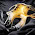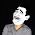## Wednesday, November 30, 2011

### Expressions and Operators in ActionScript 3

An expression is something that computes or evaluates into a single value. In a very simple form, a reference to a variable is already an expression. For example:

``````var quantity:Number = 5;
trace(quantity);``````

Here, the variable quantity evaluates to a value of 5.

An expression can also be made up of multiple values that are manipulated in some kind of operation. For example:

``trace(10 + 5);``

Here, we have two values: 10 and 5. The values in this expression are referred to as operands. These operands are going to be added together, as signified by the plus symbol. The plus symbol is referred to as the operator in this expression. Operators are special characters that are used to perform operations between values or operands. And once the operation in this example is complete, the expression evaluates to a value of 15.

Let's take a look at some of the operators you can use in ActionScript 3.

Arithmetic Operators
• Plus (+)
• Minus (-)
• Multiply (*)
• Divide (/)
• Modulo (%)

Examples:
``````trace(10 + 5);
trace(5 - 3);
trace(2 * 2);
trace(8 / 4);``````

The plus, minus, multiply, and divide operators are pretty self-explanatory. As for the Modulo operator, this will return the remainder from a division operation.

Example:
``trace(7 % 2);``

7 divided by 2 is equal to 3 remainder 1. A modulo operation will return the remainder, so 7 % 2 will return 1.

When performing an arithmetic operation on expressions that have more than two different operators, use parentheses to group the operands and operators that you would like to compute for first.

Example:
``````var nCompute:Number = (4 + 20) / (3 * 2);
trace(nCompute);``````

In this example, the operations within the parentheses will be computed first, and then the results will be divided.

Without the parentheses, the division and multiplication operations will be calculated first, followed by the addition operation, resulting in a different value.

So the following expression, which doesn't make use of parentheses, will actually have a different outcome even though it uses the same values:
``````var nCompute2:Number = 4 + 20 / 3 * 2;
trace(nCompute2);``````

Assignment Operators
• Assignment (=)
• Subtraction Assignment (-=)
• Multiplication Assignment (*=)
• Division Assignment (/=)

Let's take a look at the Assignment operator first. The Assignment operator is represented by the equals sign (=). It can be used to assign a value to an operand.

Example:
``var nScore:Number = 10;``

In this example, the Assignment operator is used to assign a value of 10 to the nScore variable. The operand to the right of the equals sign is assigned to the operand to the left of the equals sign.

The other assignment operators (Addition Assignment, Subtraction Assignment, Multiplication Assignment, and Division Assignment) allow you to write shorthand versions of arithmetic and assignment operations combined.

Example:
``````var nScore:Number = 10;
trace(nScore += 5);
// Outputs a value of 15``````

In this example, nScore += 5 is just the shorthand version of writing nScore = nScore + 5. This means that you are adding 5 to whatever the current value of nScore is.

Comparison Operators
• Equality (==)
• Inequality (!=)
• Greater than (>)
• Less than (<)
• Greater than or equal (>=)
• Less than or equal (<=)

Comparison operators are used to compare values. An expressions that uses comparison operators will evaluate to either true or false.

Examples:
``````trace(4 == 4);
// This expression tests if 4 is equal to 4.
// This evaluates to true since 4 really is equal to 4.

trace(6 != 6);
// This expression tests if 6 is NOT equal to 6.
// This evaluates to false since the two operands
// have the same value and are therefore equal.

trace(10 > 7);
// This expression tests if 10 is greater than 7.
// Since 10 really is greater than 7,
// then this expression evaluates to true.``````

Logical Operators
• And (&&)
• Or (||)

The And and Or logical operators allow you to combine two or more sets of comparison expressions.

If you use the And operator, the comparison expressions you are combining ALL have to be true in order for the entire expression to return a value of true.

Examples:
``````trace(4 == 4 && 5 == 5);
// This evaluates to true since both comparison expressions are true.
// 4 is equal to 4 AND 5 is equal to 5.

trace(4 == 4 && 5 == 6);
// This evaluates to false. Even if 4 is equal to 4,
// the second comparison expression is false;
// So the whole thing becomes false.``````

If you use the Or operator, only one of the expressions needs to be true in order to return a value of true.

Example:
``````trace(4 == 4 || 5 == 6);
// This still evaluates to true.
// Even if the second comparison expression is false,
// the first one is still true, so the whole expression
// evaluates to true.``````

The Not Operator
• Not (!)

The Not operator expresses the opposite of a Boolean.

Example:
``````var compare:Boolean = 10 < 5;
// This evaluates to false since 10 is, in fact,
// not less than 5.

trace(!compare);
// This will output true.``````

The value of compare is false. In the trace statement however, the variable is being negated by the Not operator, so the trace statement is actually going to output the opposite of false, which is true.

The Concatenation Operator

The Concatenation operator, which also uses the plus (+) sign, allows you to concatenate or combine String values.

Example:
``````var sFName:String = "John";
var sLName:String = "Doe";
trace(sFName + " " + sLName);
// This outputs John Doe``````

In this example, the Concatenation operator combines the String values together. In between sFName and sLName are two quotation marks with nothing but a single empty space inside. This is an empty string that just adds a space in between the other two String values.

The Increment and Decrement Operators
• Increment (++)
• Decrement (--)

The Increment operator increments a numeric value by one (plus 1). The Decrement operator decrements a numeric value by one (minus 1).

Example:
``````var nScore:Number = 10;
nScore++;
trace(nScore);
// Outputs 11 (10 + 1)``````

In this example, nScore++ is the same as writing nScore = nScore + 1 or nScore += 1.

NOTE: If you try to trace nScore++ immediately like so:
var nScore:Number = 10;
trace(nScore++);
This will still output a value of 10. When using the increment and decrement operators, the variable will be traced first, so the original value will come out. Only after that will it be incremented or decremented. To avoid that, you can put the operator before the variable like so:
trace(++nScore);

And that concludes this lesson on expressions and operators in ActionScript 3.

1.This is a lot to digest, but good stuff. Maybe add in some real world uses and lesson examples to re-inforce learning. Cheers

2.Nicely described

3.Very Nice tut... Thanks a lot!!!! Appreciate the way you explained all easily..

4.and thanks for providing it free of cost...

5.Thanks a lot for these. Especially since they are free.

6.Very Nice tutorial

7.Well Illustrated..
(kishor)

8.good useful for me

9.awesome writer made an awsome article